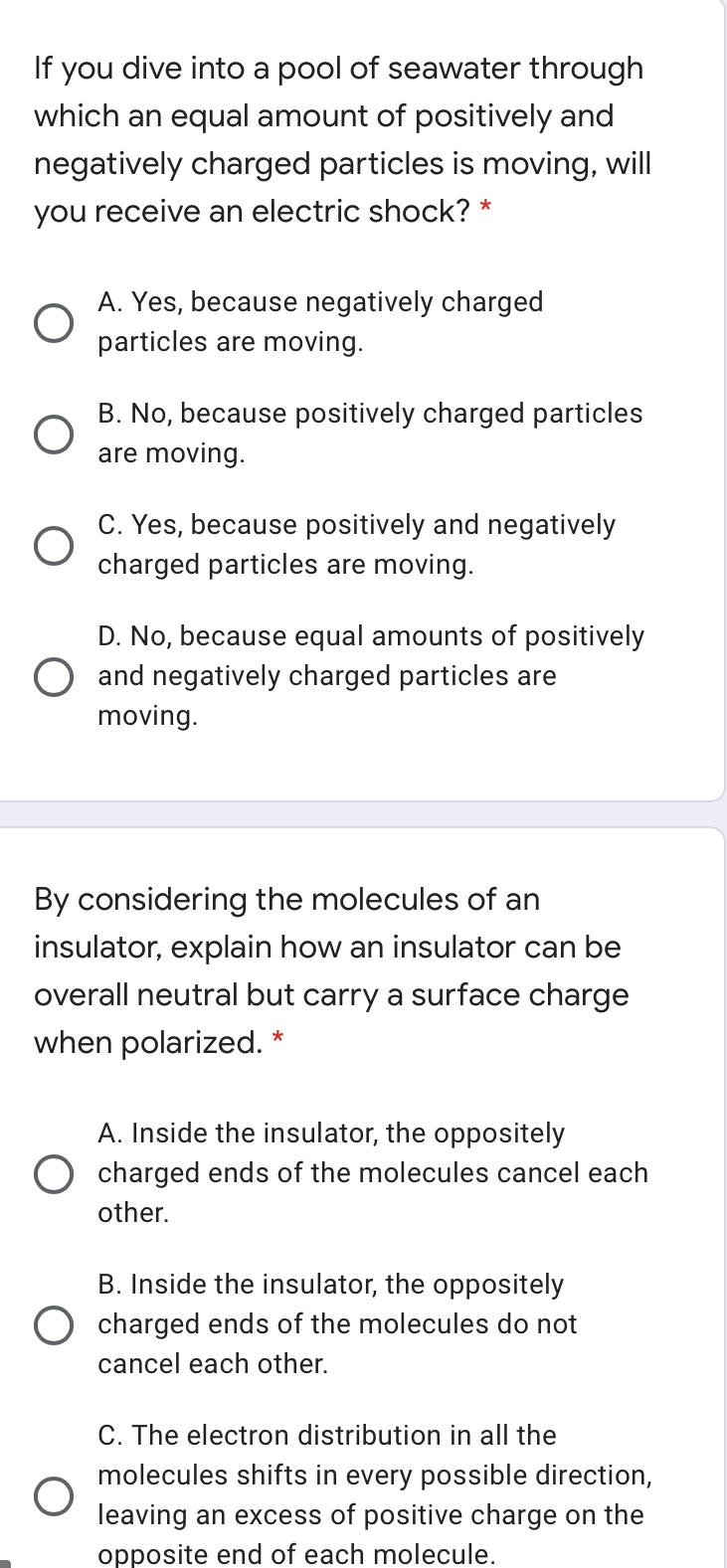Question:

# If you dive into a pool of seawater through which an equal amount of positively and negatively charged particles is moving, willIf you dive into a pool of seawater through which an equal amount of positively and negatively charged particles is moving, will you receive an electric shock? * O A. Yes, because negatively charged particles are moving. B. No, because positively charged particles are moving. C. Yes, because positively and negatively charged particles are moving. D. No, because equal amounts of positively O and negatively charged particles are moving. By considering the molecules of an insulator, explain how an insulator can be overall neutral but carry a surface charge when polarized. * A. Inside the insulator, the oppositely charged ends of the molecules cancel each other. B. Inside the insulator, the oppositely charged ends of the molecules do not cancel each other. O C. The electron distribution in all the molecules shifts in every possible direction, leaving an excess of positive charge on the opposite end of each molecule.